Mobile QR Code1. (Department of Electrical Engineering, Pusan National University, Busan 46241, Korea)
2. (Department of Information and Communication Engineering, Hanbat National University, Daejeon 34158, Korea)

## I. INTRODUCTION

Quadrature couplers have been widely used in design of balanced amplifiers, phase shifters, and balanced mixers, as well as other microwave and millimeter-wave components. Lumped-element quadrature couplers are typically used for on-chip implementations (1-6). Fig. 1 shows a schematic of a lumped-element quadrature coupler. The signal power on the input port is equally divided into direct and coupled ports with a phase difference of 90° provided that the coupling coefficient, kL, is 0.707. Design values for each element can be founded in (1). In this regard, electromagnetic (EM) simulators are widely employed to find design values of each element. Despite the large applicability of the quadrature coupler, an accurate model of lumpedelement quadrature couplers has not yet been developed, to the best of our knowledge. A less accurate model was reported for in (2), but the focus was on equation derivation, and modeled responses were not compared with measured ones. Although EM simulations provide a possible approach for the design of lumped-element quadrature coupler and for predicting the performance of the coupler, the simulation time for optimization can be long. Moreover, for getting design insight and for optimization of quadrature couplers, an accurate model is required.

One of main difficulties for the design of lumpedelement quadrature couplers, whose generic schematic is depicted in Fig. 1(b), is to achieve a high, well-controlled coupling coefficient (1,2). To obtain a high coupling coefficient, the number of turns in inductors typically needs to be increased in a lateral configuration. It causes the degradation of the quality factor of inductors, resulting in degradation of insertion loss and phase balance performance (1,3,4).

In this work, we propose an accurate distributed model for a lumped-element quadrature coupler based on physical geometry. In addition, by adopting the proposed distributed model, a low-loss coupler with a relatively low coupling coefficient was implemented. Its results are compared with modeled responses.

Fig. 1. (a) Generic four-port quadrature coupler, (b) schematic of lumped-element quadrature coupler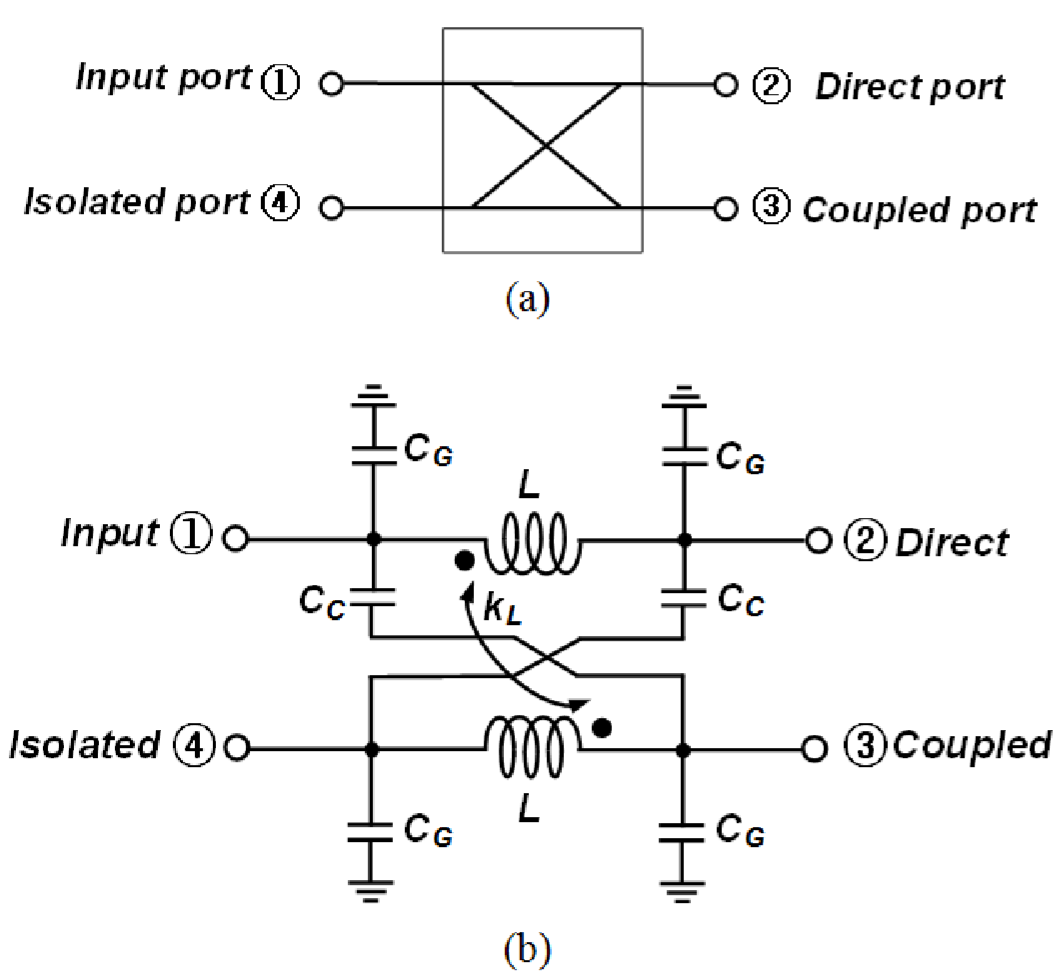## II. COUPLER MODEL AND DESIGN OF LOW LOSS QUADRATURE COUPLER

A lumped-element quadrature coupler, which is shown in Fig. 1(b), is also called Langer coupler because it employs coupled inductors in its geometry. The equation for the design parameters of the lumped-element quadrature coupler in Fig. 1(b) can be derived by equating with the parameters of the transmission-line coupler. In (1), the design values of each parameter can be founded by the following equation:

##### (1)
$L=\frac{1.414 \times Z_{0}}{\omega_{0}}$ $C_{C}=\frac{1}{\omega_{O} Z_{0}},$ and $C_{G}=\frac{0.414}{\omega_{O} Z_{0}}$

where ω0 is the operating frequency and Z0 is the characteristic impedance. At 5.5 GHz, the parameter values of Fig. 1(b) are set as follows: $L$ = 2.05 nH, CC = 0.58 pF, CG = 0.24 pF, and kL = 0.707.

For the design of the coupled inductors, to achieve a large coupling coefficient of 0.707, the number of turns is typically increased in a lateral configuration, resulting in the decrease of the quality factor of the inductor, $Q$. Fig. 2 shows the plots of simulated S-parameter characteristics with different $Q$ values using an Advanced Design System (ADS) simulator. Low $Q$ will degrade the insertion loss as well as the phase balance performance (1,3). Although the adjustment for values of CC and CG can be performed for better characteristic (1), it can disrupt the symmetry of the coupler and there is no formal analysis for this optimization process (1).

Fig. 2. Simulated S-parameter characteristics with different $Q$ values (a) insertion losses, (b) phase differences, (c) isolations, (d) return losses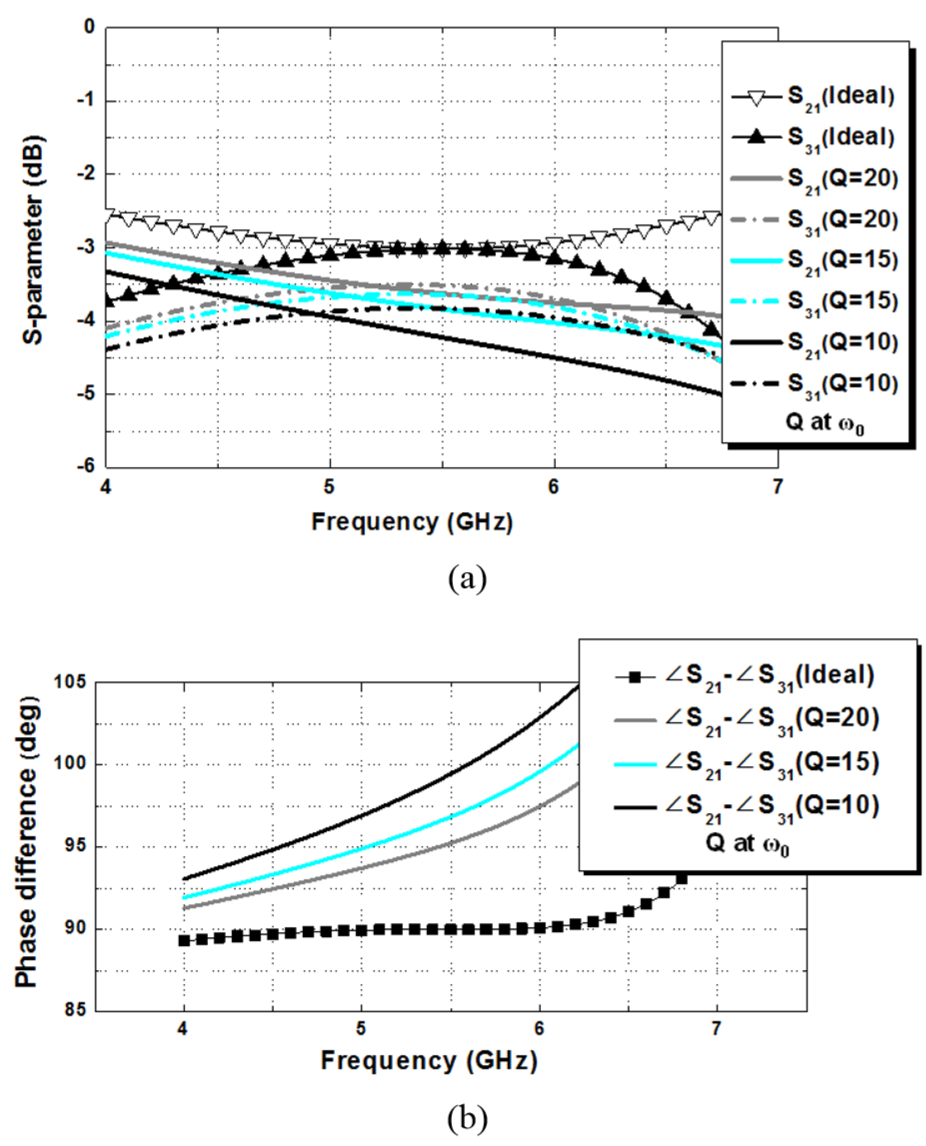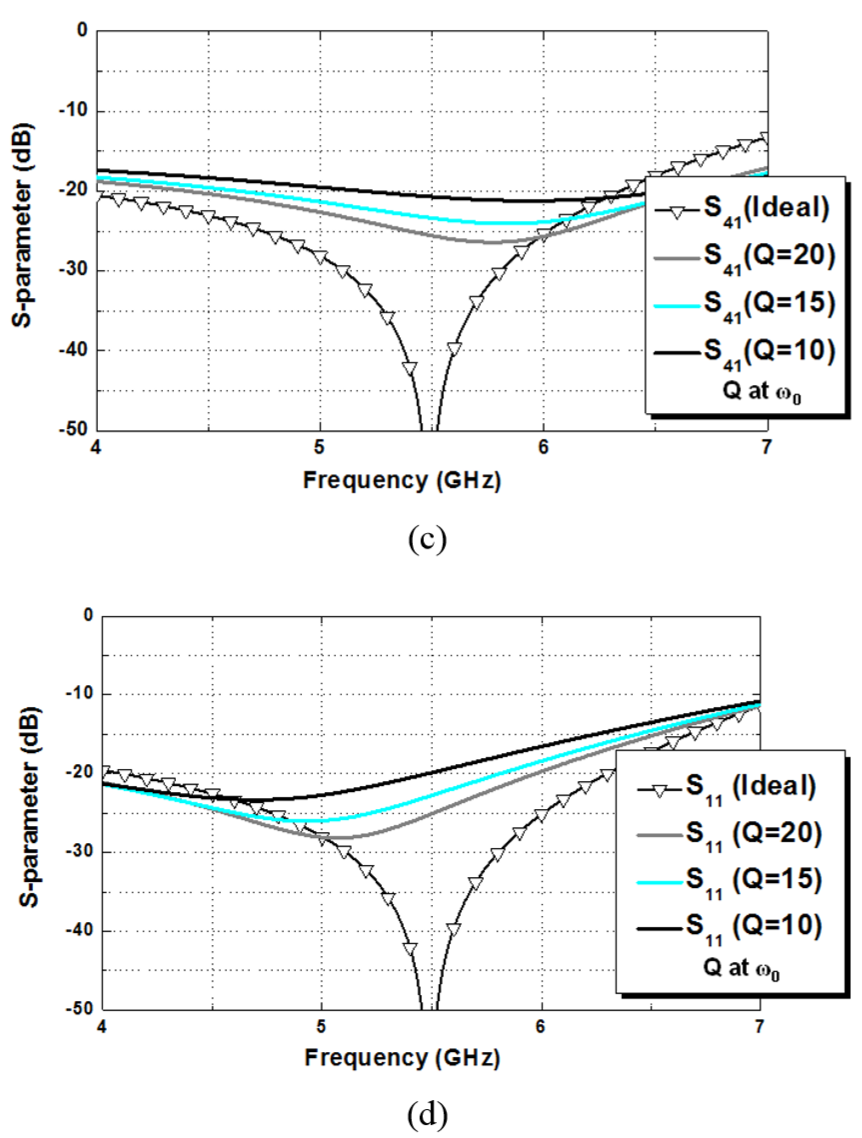### 2. Quadrature Coupler using Distributed Model

The key to achieve an accurate modeling of couplers is the identification of relevant parasitic components. Unlike models of inductors or transformers, the composition of parasitic capacitance in lumped-element couplers plays a crucial role for the normal operation of quadrature couplers. To accurately model the composition of parasitic capacitance, distributed effects should be carefully considered.

Fig. 3(a) shows a layout of a symmetrical quadrature coupler. Two metal traces are inter-winded together. Each winding is referred to as a 1.5-turn inductor. A topmost thickest metal layer is used for most inductor winding to minimize its loss. A lower metal layer is used only for underpass elements. Each metal winding is divided into three segments, as shown in Fig. 3(a). The proposed equivalent circuit model for the quadrature coupler is depicted in Fig. 3(b). Each segment is generated independently, but then linked together with additional mutual capacitive and magnetic coupling components. Each segment includes self-inductance, $L$, with ZS. The series resistance, Rsm, in ZS represents a series metal loss. ZS also includes L-R branches, Rpm, and Lpm, to model skin and proximity effects. At starting and ending points of each segment, parasitic capacitances are considered. External capacitors for CC and CG in Fig. 1(b) can be added. However, because parasitic capacitances from two metal traces can be absorbed for a large portion of the required CC and CG in Fig. 1(b), they should be carefully analyzed. Considering coupler design using GaAs technology, CG is a parasitic capacitance of dielectric and substrate owing to the absence of resistance in a semiinsulating GaAs substrate. Fig. 3(a) also shows the metal stack-up information of a GaAs technology. The mutual magnetic coupling between two adjacent inductors is indicated with the coupling coefficient, kL. Note that CC in Fig. 3(a) describes the coupling capacitance between two adjacent segments. Given that the spacing is typically required to be small to increase kL and the metal thickness is usually larger than 3 μm in most CMOS and GaAs technology, the parasitic CC can constitute a large portion of the required total CC. Note that Kp is the additional coupling coefficient between segments in the same winding.

### 3. Design of Low Loss Quadrature Coupler

Lumped models in light yellow and light green blocks in Fig. 3(b) are identical for an ideal quadrature coupler if CG and CC at middle point are neglected. It seems that two ideal lumped-element couplers are combined in series. In (3,4), the required coupling coefficient in a two-stage cascade design of quadrature coupler can be much lower than that of an ideal single-stage coupler. A coupler design with high $Q$ will improve its loss performance. Therefore, in this study, a low-loss quadrature coupler is developed with a relatively low coupling coefficient using the proposed model. The proposed quadrature coupler structure using a distributed model includes loss resistors, as shown in Fig. 3(b). Thus, unlike coupler designs using (1), the design method using the proposed model can be optimized with finite $Q$ of inductors. In addition, because a cascade structure has a relatively large bandwidth performance, the model in Fig. 3(b) with a structure similar to a cascade can improve the bandwidth performance.

## III. IMPLEMENTATION AND MEASUREMENTS

The proposed low-loss quadrature coupler was fabricated using GaAs technology. The thicknesses of the top and lower metal layers are 4 μm and 1 μm, respectively. A metal layer with a large width is used to improve $Q$. Specifically, this width is 30 μm. A spacing of 10 μm is chosen for kL and CC. The size of the coupler is mainly determined to provide the necessary inductance $L$. However, increasing the size also increases the parasitic capacitances, CC and CG. Its size is 1250 μm × 650 μm. In this design, no external capacitor is added. The values for each component in Fig. 3(b) are obtained from optimization based on the initial values from physical properties. A slight adjustment within the physical range is done for better fitting with measurement results. The value of kL in Fig. 3(b) is 0.51, which is lower than the value from an ideal lumped- element quadrature coupler. Fig. 4 shows the microphotograph of the fabricated quadrature coupler.

The S-parameter on-wafer measurement was carried out using a four-port Agilent N5242A network analyzer and Cascade S300 probe station. During the procedure in full four-port calibration, calibration data was measured by connecting an open standard, a short standard, a load standard, and a through standard to the four test ports. After the calibration process, the integrated coupler was measured. The measured insertion losses between input and direct ports (S21) and between input and coupled ports (S31) are compared with the modeled responses. As shown in Fig. 5, the modeled responses are well matched with the measured responses. The measured insertion losses for both S21 and S31 are 3.23 dB at 5.5 GHz. Across a 760-MHz bandwidth, the insertion loss varies only ± 0.3 dB. Fig. 6 shows the phase differences between S21 and S31 in the measured and modeled responses. The measured phase difference is less than 0.95° over a 760MHz bandwidth. Fig. 7 and 8 show the responses of isolations and return losses of the implemented coupler, respectively. The measured isolation and return losses are less than 30 dB and 23 dB across a 760-MHz bandwidth, respectively. There is a slight gap between modeled and measured responses. The values for inductors and capacitors of each segment are slightly different each other owing to the different length of each element, which can give rise to discrepancies between modeled and measured responses. However, the same values for the components in each segment are applied to provide a design insight. The results of the proposed quadrature coupler are compared in Table 1 with previously published results. The proposed coupler achieves lower insertion loss with low phase error over a wide bandwidth compared with other works.

Fig. 4. Microphotograph of the integrated quadrature couplerFig. 5. Measured and modeled insertion losses of quadrature coupler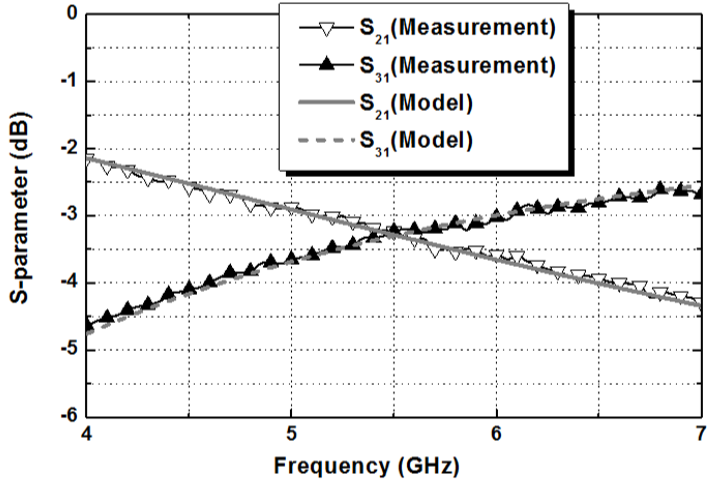Fig. 6. Measured and modeled phase differences of quadrature coupler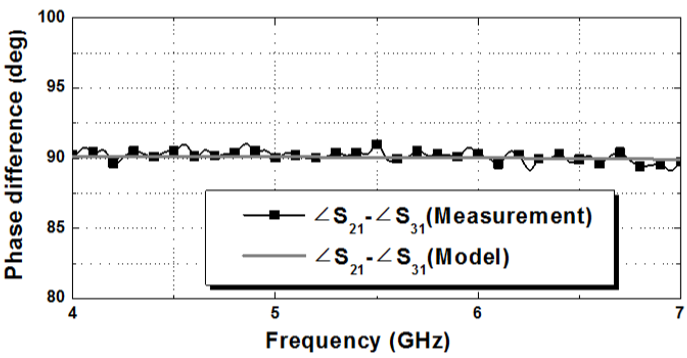Fig. 7. Measured and modeled isolations of quadrature coupler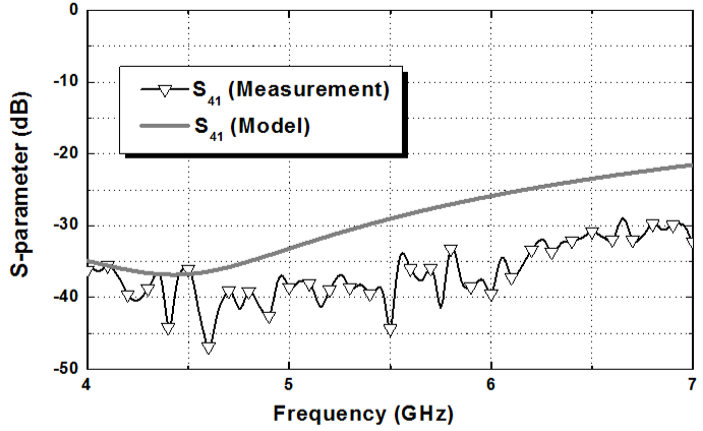Fig. 8. Measured and modeled return losses of quadrature coupler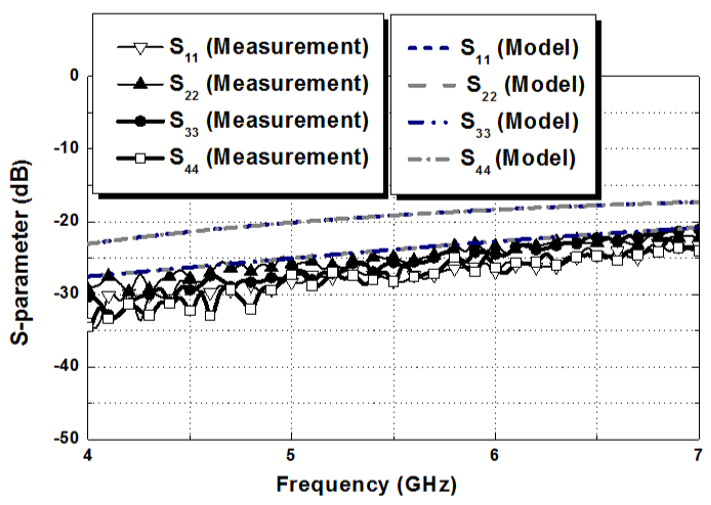Table 1. Comparison table with integrated quadrature coupler

 Ref. (3) (5) (6) This work Tech. CMOS CMOS GaAs GaAs Frequency range (GHz) 5.4 4.2 5.2 5.5 Bandwidth (MHz) (Fractional BW) 400 (7%) 400 (10%) 100 (2%) 760 (13.8%) Loss (dB) 5.0 ± 0.2 3.7 ± 0.3 3.5 ± 0.3 3.23 ± 0.3 Phase Balance (deg) 0.86 ± 0.7° 3.5° 6° 0.95° Isolation (dB) 21 14 27 30 Return loss (dB) 18 15 22 23 Power Consump. (mW) 0 20.4 0 0

## IV. CONCLUSIONS

An accurate distributed model for a quadrature coupler has been proposed. The proposed approach is validated with measured results. The model agrees well with measured results. This work shows that couplers with the proposed accurate model can achieve low insertion loss across a wide bandwidth. The proposed distributed model can be directly applied to the design of any lumpedelement quadrature couplers.

### ACKNOWLEDGMENTS

This work was supported by Korea Institute for Advancement of Technology(KIAT) grant funded by the Korea Government(MOTIE) (P078200021, The Competency Development Program for Industry Specialist). The CAD tools were supported by the IDEC.

### REFERENCES

1
Frye R. C., Kapur S., Melville R. C., Oct 2003, A 2-GHz quadrature hybrid implemented in CMOS technology, IEEE J. Solid-State Circuits, Vol. 38, No. 3, pp. 550-5552
Chin T.-Y., Wu J.-C., Chang S.-F., Chang C.-C., Mar 2009, Compact S-/Ka-band CMOS quadrature hybrids with high phase balance based on multilayer transformer over-coupling technique, IEEE Trans. Microw. Theory Tech., Vol. 57, No. 3, pp. 708-7153
Ozis D., Allstot D. J., Jan 2006, A CMOS 5 GHz phase-compensated quadrature coupler, in IEEE Radio Wireless Symp., pp. 51-544
Ozis D., Paramesh J., Allstot D. J., May 2009, Integrated quadrature couplers and their application in image-reject receivers, IEEE J. Solid-State Circuits, Vol. 44, No. 5, pp. 1464-14765
Hsieh H.-H., Liao Y.-T., Lu L.-H., Jun 2007, A compact quadrature hybrid MMIC using CMOS active inductors, IEEE Trans. Microw. Theory Tech., Vol. 55, No. 6, pp. 1098-11046
Ellinger F., Vogt R., Bachtold W., Apr 2002, Ultracompact reflective-type phase shifter MMIC at C-band with 360° phase-control range for smart antenna combining, IEEE J. Solid-State Circuits, Vol. 37, No. 4, pp. 481-486## Author

received the B.S. degree in electrical engineering from Pusan National University, Busan, Korea, in 2015, and is currently pursing the Ph.D. degree in electrical engineering at Pusan National University, Busan, Korea.

His interests include high-frequency integrated circuits and system design for wireless communications.

received the B.S. degree in EE from Yonsei University, Korea, in 1999, and the M.S. and Ph.D. degrees in EECS from the KAIST, Korea, in 2001 and 2005, respectively. From 2005 to 2007, he was a Senior Engineer with Samsung Electronics, Gyeonggi, Korea, where he was involved in the development of mobile digital TV tuner IC.

In 2007, he joined the School of Electrical Engineering, Pusan National University, Busan, Korea, and is now a Professor.

received the B.S., M.S., and Ph.D. degrees in electrical engineering from Korea Advanced Institute of Science and Technology (KAIST), Daejeon, Korea, in 2000, 2002, and 2007, respectively.

From 2007 to 2009, he was in Georgia Institute of Technology, where he developed CMOS power amplifiers.

In 2009, he joined Skyworks Solutions, Inc., Cedar Rapids, IA, where he was involved with the design of power amplifiers and front end modules.

In 2010, he joined the faculty of Hanbat National University, Daejeon, Korea.

His research interests include RF power amplifier design, microwave module design, and ultrasonic circuit design.

received the B.S. degree in electrical engineering from Sungkyunkwan University, Korea, in 2001, the M.S. degree in electrical engineering from the KAIST, Korea, in 2005, and the Ph.D. degree in electrical and computer engineering from the Georgia Institute of Technology, USA, in 2009.

Upon completion of the doctoral degree, he joined Qualcomm Inc., USA, as a Senior Engineer, where he was involved in the development of transmitters and integrated passive circuits on mobile applications.

He is currently a faculty member with the Department of Electrical Engineering, Pusan National University, Korea.

His research interests include high-frequency integrated circuits and system design for wireless communications.